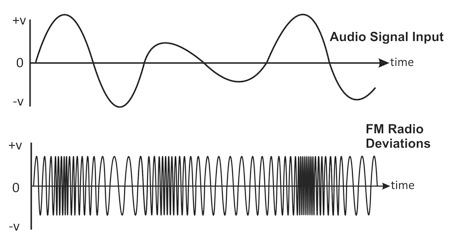top of page
Search
• Stu WØSTU

# Carson's Rule (G8B06)

The 2019-2023 General License question pool requires the use of Carson's Rule to estimate the bandwidth of an FM phone transmission:

G8B06: What is the total bandwidth of an FM-phone transmission having a 5 kHz deviation and a 3 kHz modulating frequency?

A. 3 kHz B. 5 kHz C. 8 kHz D. 16 kHz

Let’s review some FM basics first, and then we’ll jump into this calculation that is an application of Carson’s Rule for estimating FM signal maximum bandwidth.

Frequency modulation (FM) encodes information, such as a voice, into the RF signal by deviating the transmission frequency about a known reference frequency, the carrier frequency. Put a slightly different way, the FM carrier is deviated from its resting frequency when the modulating signal is applied to the FM transmitter. Further, the magnitude of the deviation is proportional to the instantaneous amplitude of the modulating audio signal. Here is a notional depiction of frequency deviations resulting from amplitude variations in a driving audio signal.Frequency deviations in an FM signal are determined by the amplitude of the modulating signal.

Notice that as the audio signal input amplitude increases to a positive voltage value the frequency of the RF carrier gets higher. As the amplitude of the audio signal input goes to a negative voltage the frequency of the RF carrier gets lower. The FM carrier bounces back and forth across a range of RF frequencies proportional to the amplitude of the audio signal. (And also at a deviation rate equivalent to the frequency of the audio signal.)

As the amplitude of a driving audio signal increases the FM deviation will occupy a broader swath of frequencies commensurate with that greater audio amplitude. So, talk louder into your microphone and your FM signal will increase in RF bandwidth in order to represent that increased audio amplitude with greater deviations. Talk softly for a reduced amplitude audio signal and the RF deviations are commensurately less extreme, occupying less bandwidth.

FM signals will have variable bandwidth depending upon the amplitude of the audio input signal, and also depending upon other characteristics of the transmitter and the modulating signal.

You should now ask, “What other characteristics impact FM bandwidth?” You might guess from the formulation of question G8B06: 1) the peak deviation, and 2) the highest frequency of the modulating (audio) signal. The peak deviation is the greatest RF carrier deviation allowed by the transmitter circuits, and the highest modulating frequency is the highest audio frequency transferred by the microphone, audio amplifier, and other circuits into the FM transmitter.

In 1922 John Renshaw Carson formulated his rule for estimating the bandwidth of FM signals using these two parameters. Since the actual bandwidth of the FM signal will vary with the modulating signal amplitude, Carson’s Rule is an estimation of the maximum bandwidth that an FM signal will occupy. Thus, an FM signal will vary in bandwidth commensurate with the audio signal amplitude, but with a maximum bandwidth estimated by Carson’s simple equation, as follows:

FM Bandwidth = 2(Δf + fm),

where Δf is the peak frequency deviation and fm is the highest modulating frequency.

Now we can compute an estimate for the bandwidth of the question’s transmission, with a peak deviation of 5 kHz and a modulating high frequency of 3 kHz:

FM Bandwidth = 2(5 kHz + 3 kHz) FM Bandwidth = 2(8 kHz) FM Bandwidth = 16 kHz

The 5 kHz peak deviation referenced in this question is considered “standard FM” on the US VHF and UHF amateur bands. Further, a typical modulating audio signal generated from a human voice will have a high frequency of around 3 kHz. Thus, this question’s example is representative of a typical voice FM signal in the US.

Some amateur transmitters provide a 2.5 kHz peak deviation option, thereby generating a narrower bandwidth FM signal. For instance, given the same high modulating frequency of 3 kHz, a 2.5 kHz peak deviation results in a bandwidth of 11 kHz, in accordance with Carson’s Rule. Although rarely used in the US, “narrow FM” is used in the UK. The narrower bandwidth signals provide for more efficient use of available RF spectrum.

The answer to General Class question G8B06, What is the total bandwidth of an FM-phone transmission having a 5 kHz deviation and a 3 kHz modulating frequency? is “D. 16 kHz.”

-- Stu WØSTU

2,216 views

See All
bottom of page